Mar 11, 2021

# How to tune hyperparams with fixed seeds using PyTorch Lightning and Aim

Author: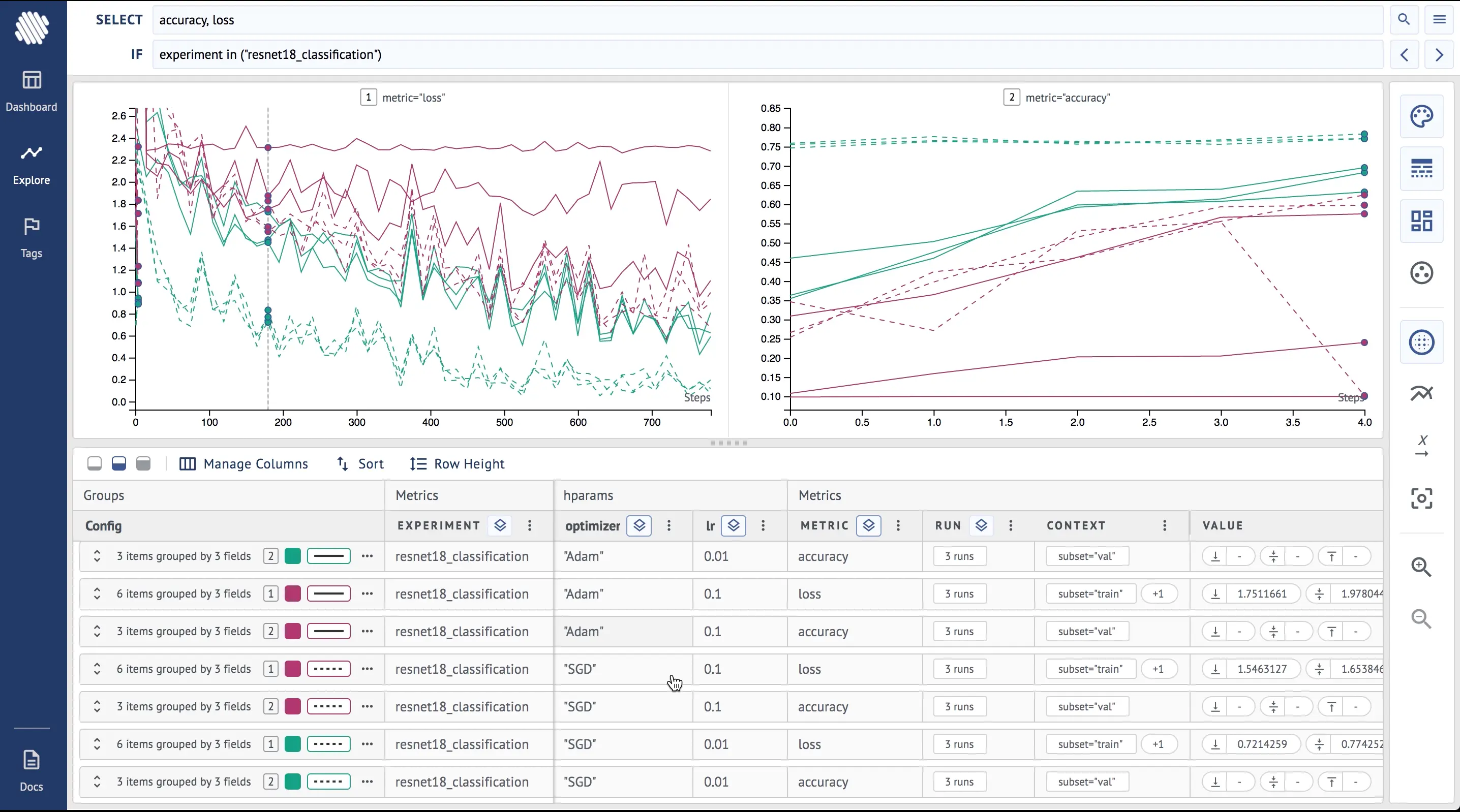## What is a random seed and how is it important?

The random seed is a number for initializing the pseudorandom number generator. It can have a huge impact on the training results. There are different ways of using the pseudorandom number generator in ML. Here are a few examples:

• Initial weights of the model. When using not fully pre-trained models, one of the most common approaches is to generate the uninitialized weights randomly.
• Dropout: a common technique in ML that freezes randomly chosen parts of the model during training and recovers them during evaluation.
• Augmentation: a well-known technique, especially for semi-supervised problems. When the training data is limited, transformations on the available data are used to synthesize new data. Mostly you can randomly choose the transformations and how there application (e.g. change the brightness and its level).

As you can see, the random seed can have an influence on the result of training in several ways and add a huge variance. One thing you do not need when tuning hyper-parameters is variance.

The purpose of experimenting with hyper-parameters is to find the combination that produces the best results, but when the random seed is not fixed, it is not clear whether the difference was made by the hyperparameter change or the seed change. Therefore, you need to think about a way to train with fixed seed and different hyper-parameters. the need to train with a fixed seed, but different hyper-parameters (comes up)?.

Later in this tutorial, I will show you how to effectively fix a seed for tuning hyper-parameters and how to monitor the results using Aim.

## How to fix the seed in PyTorch Lightning

Fixing the seed for all imported modules is not as easy as it may seem. The way to fix the random seed for vanilla, non-framework code is to use standard Python`random.seed(seed)`, but it is not enough for PL.

Pytorch Lightning, like other frameworks, uses its own generated seeds. There are several ways to fix the seed manually. For PL, we use `pl.seed_everything(seed)` . See the docs here.

Note: in other libraries you would use something like: `np.random.seed()` or `torch.manual_seed()`

## Implementation

Find the full code for this and other tutorials here.

``````import torch
from torch.nn import functional as F
from torchvision.datasets import CIFAR10
from torchvision.models import resnet18
from torchvision import transforms
import pytorch_lightning as pl
from aim.pytorch_lightning import AimLogger

class ImageClassifierModel(pl.LightningModule):

def __init__(self, seed, lr, optimizer):
super(ImageClassifierModel, self).__init__()
pl.seed_everything(seed)
# This fixes a seed for all the modules used by pytorch-lightning
# Note: using random.seed(seed) is not enough, there are multiple
# other seeds like hash seed, seed for numpy etc.
self.lr = lr
self.optimizer = optimizer
self.total_classified = 0
self.correctly_classified = 0
self.model = resnet18(pretrained = True, progress = True)
self.model.fc = torch.nn.Linear(in_features=512, out_features=10, bias=True)
# changing the last layer from 1000 out_features to 10 because the model
# is pretrained on ImageNet which has 1000 classes but CIFAR10 has 10
self.model.to('cuda') # moving the model to cuda

train_ds = CIFAR10('.', train = True, transform = transforms.ToTensor(), download = True)

val_ds = CIFAR10('.', train = False, transform = transforms.ToTensor(), download = True)

def forward(self, x):
return self.model.forward(x)

def training_step(self, batch, batch_nb):
x, y = batch
y_hat = self.model(x)
loss = F.cross_entropy(y_hat, y)
# calculating the cross entropy loss on the result
self.log('train_loss', loss)
# logging the loss with "train_" prefix
return loss

def validation_step(self, batch, batch_nb):
x, y = batch
y_hat = self.model(x)
loss = F.cross_entropy(y_hat, y)
# calculating the cross entropy loss on the result
self.total_classified += y.shape
self.correctly_classified += (y_hat.argmax(1) == y).sum().item()
# Calculating total and correctly classified images to determine the accuracy later
self.log('val_loss', loss)
# logging the loss with "val_" prefix
return loss

def validation_epoch_end(self, results):
accuracy = self.correctly_classified / self.total_classified
self.log('val_accuracy', accuracy)
# logging accuracy
self.total_classified = 0
self.correctly_classified = 0
return accuracy

def configure_optimizers(self):
# Choose an optimizer and set up a learning rate according to hyperparameters
elif self.optimizer == 'SGD':
else:
raise NotImplementedError

if __name__ == "__main__":

seeds = [47, 881, 123456789]
lrs = [0.1, 0.01]

for seed in seeds:
for optimizer in optimizers:
for lr in lrs:
# choosing one set of hyperparaameters from the ones above

model = ImageClassifierModel(seed, lr, optimizer)
# initializing the model we will train with the chosen hyperparameters

aim_logger = AimLogger(
experiment='resnet18_classification',
train_metric_prefix='train_',
val_metric_prefix='val_',
)
aim_logger.log_hyperparams({
'lr': lr,
'optimizer': optimizer,
'seed': seed
})
# initializing the aim logger and logging the hyperparameters

trainer = pl.Trainer(
logger=aim_logger,
gpus=1,
max_epochs=5,
progress_bar_refresh_rate=1,
log_every_n_steps=10,
check_val_every_n_epoch=1)
# making the pytorch-lightning trainer

trainer.fit(model)
# training the model``````

## Analyzing the Training Runs

After each set of training runs you need to analyze the results/logs. Use Aim to group the runs by metrics/hyper-parameters (this can be done both on Explore and Dashboard after Aim 1.3.5 release) and have multiple charts of different metrics on the same screen.

Do the following steps to see the different effects of the optimizers

• Go to dashboard, explore by experiment
• Add loss to `SELECT` and divide into subplots by metric
• Group by experiment to make all metrics of similar color
• Group by style by optimizer to see different optimizers on loss and accuracy and its effects

Here is how it looks on Aim: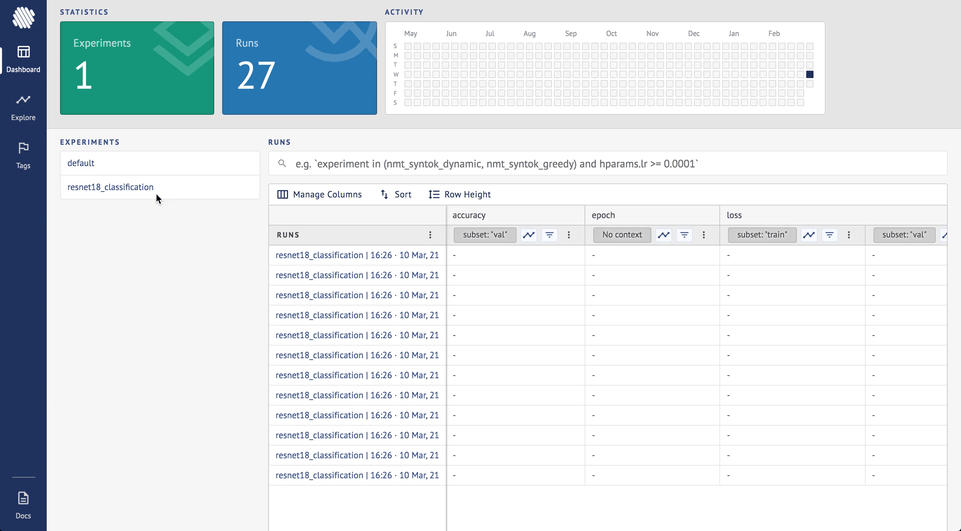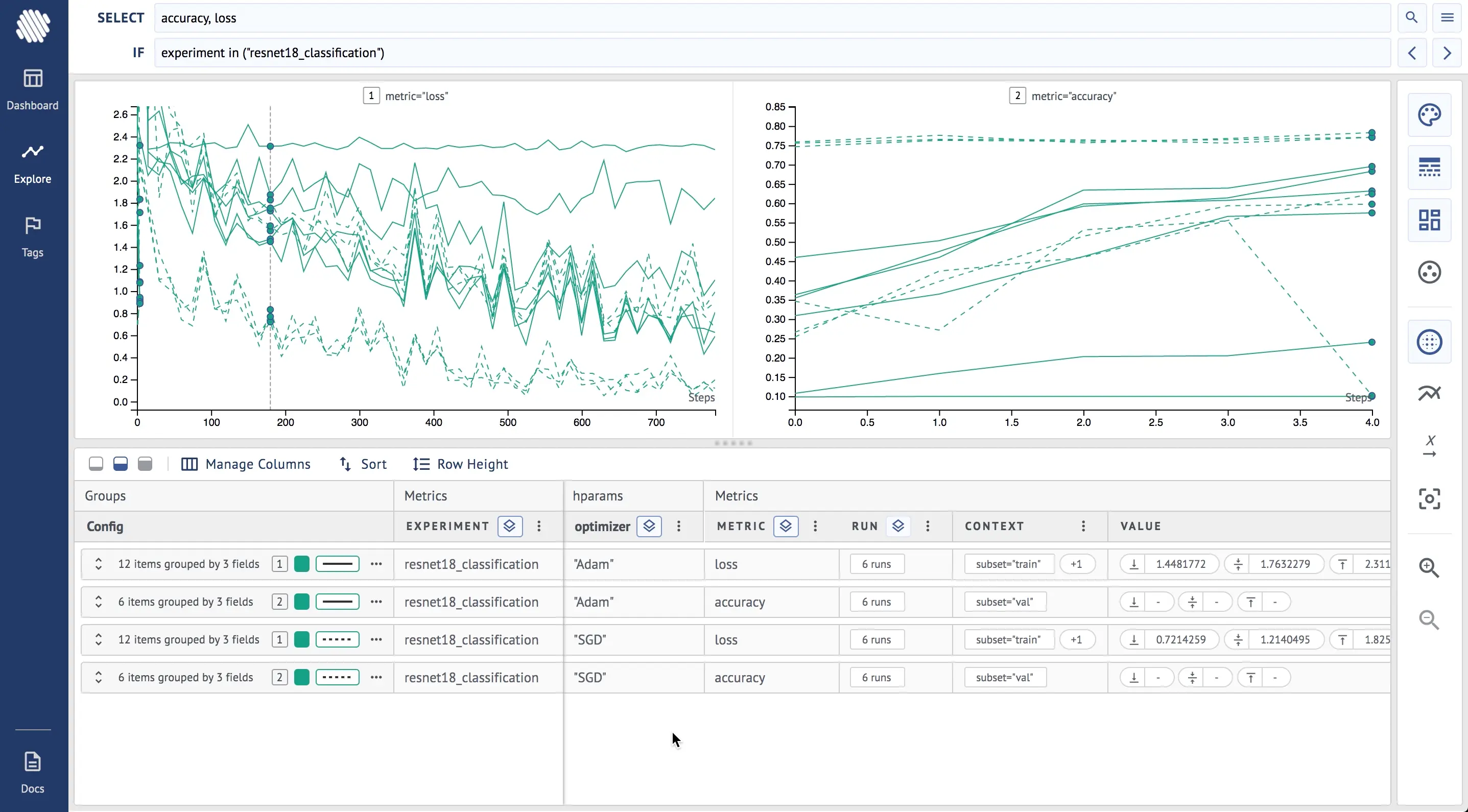From the final result it is clear that the SGD (broken lines) optimizer has achieved higher accuracy and lower loss during the training.

If you apply the same settings to the learning rate, this is the result:

## For the next step to analyze how learning rate affects the experiments, do the following steps:

• Remove both previous groupings
• Group by color by learning rate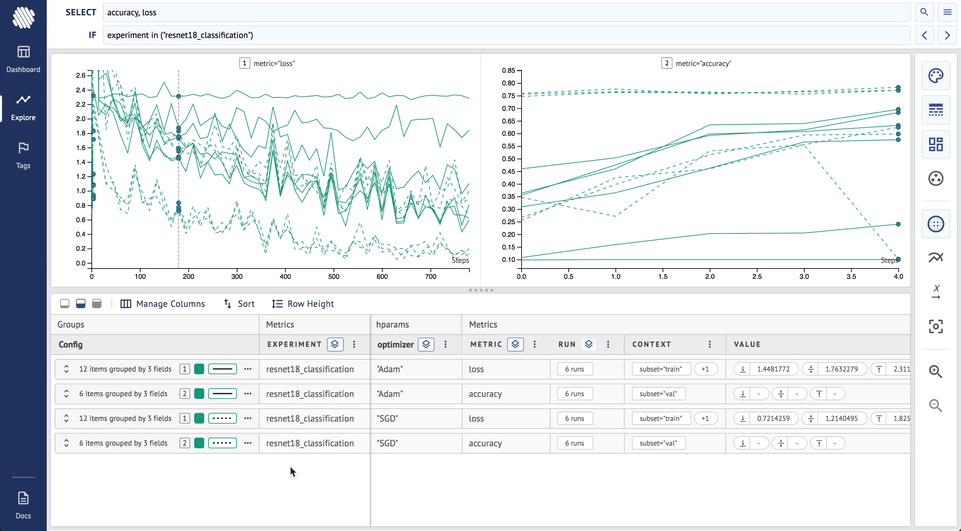As you can see, the purple lines (lr = 0.01) represent significantly lower loss and higher accuracy.

We showed that in this case, the choice of the optimizer and learning rate is not dependent on the random seed and it is safe to say that the SGD optimizer with a 0.01 learning rate is the best choice we can make.

On top of this, if we also add grouping by style by optimizer: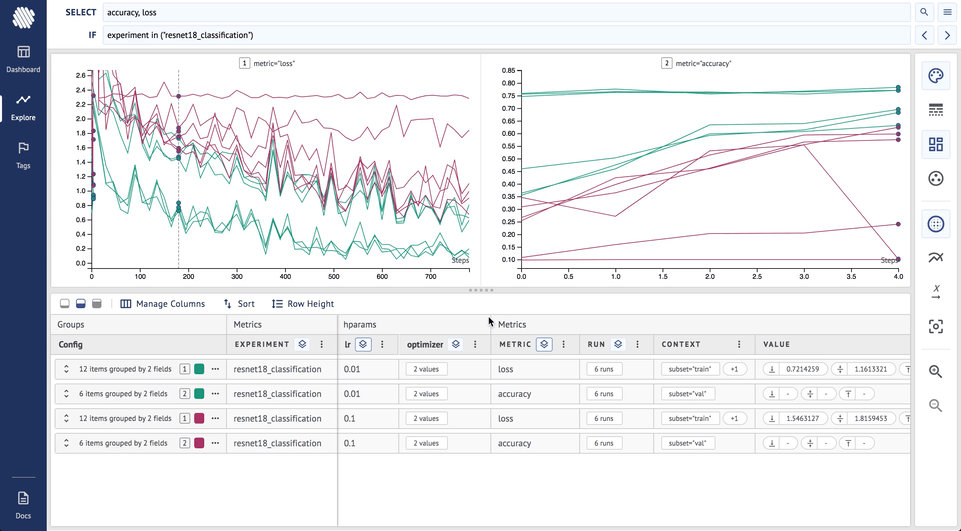Now, it is obvious that the the runs with SGD optimizer and `lr=0.01` (green, broken lines) are the best choices for all the seeds we have tried.

Fixing random seeds is a useful technique that can help step-up your hyper-parameter tuning. This is how to use Aim and PyTorch Lightning to tune hyper-parameters with a fixed seed.